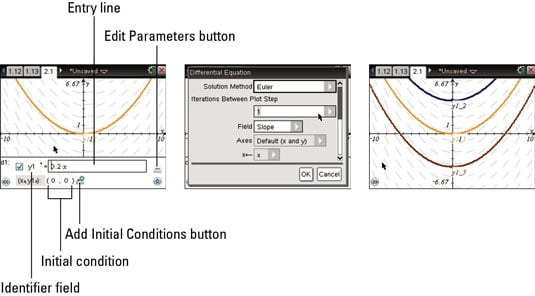##### TI-Nspire For DummiesGraphing differential equations is new feature on TI-Nspire. You can set the initial condition(s), customize the slope field, and choose your solution method (Euler or Runge-Kutta). Oh, yeah, and you can grab the initial condition and change it right on the graph screen.

Follow these steps to graph a differential equation:

This gives you a fresh start; no variables carry over.

3. Type the differential equation, y1= 0.2x2.

The default identifier is y1. To change the identifier, click the box to the left of the entry line. You may reference the identifier in the entry line.

4. Enter an initial condition, (0,0), as an ordered pair.

Press the Add Initial Conditions button to enter up to three additional initial conditions for each differential equation.

5. Press the Edit Parameters button (. . .) if you would like to customize the differential equation any further.

6. Press [ENTER] to graph the differential equation or press the down arrow to display the next differential equation edit field.Differential equation settings can be accessed by pressing the Edit Parameters button (. . .). Here is a brief summary of the settings:

• Solution Method: You have a choice of using Euler or Runge-Kutta as the numerical solution method.

• Iterations between plot step: If you are using the Euler solution method, type an integer to set the computational accuracy.

• Error Tolerance: If you are using the Runge-Kutta solution method, type a number that is greater than or equal to 1 x 10-14.

• Field: None— No field is plotted. Slope— Plots a slope field representing the solutions. Direction— Graphs a slope field representing the relationship between the values of two differential equations.

• Axes: Default(x and y)— Plots the x on the x-axis and the y on the y-axis. Custom— This setting lets you select the values to be plotted on each axis. The available entries are:

• x (the independent variable)

• y1 and y2 (or any other identifiers defined in the editor)

• y1’ and y2’ (or any other derivatives defined in the editor)

• Plot Start: This field determines the independent variable value where the solution plot starts.

• Plot End: This field determines the independent variable value where the solution plot stops.

• Plot Step: This field determines the increment of the independent variable where the values are plotted.

• Field Resolution: This field determines the number of columns used to draw the slope field. You can only change this field if Field=Direction or Slope.

• Direction Field at x=: This field sets the values of the independent variable at which a direction field is drawn when plotting autonomous equations (those that refer to x). You can only change this parameter if Field=Direction.